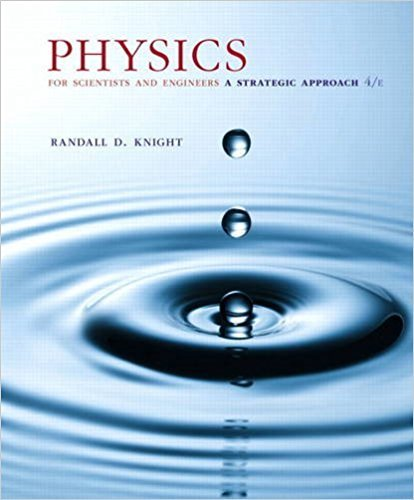×
×

# Consider a particle in a rigid box of length L. For eachISBN: 9780134081496 191

## Solution for problem 40.65 Chapter 40

Physics for Scientists and Engineers: A Strategic Approach, Standard Edition (Chs 1-36) | 4th Edition

• Textbook Solutions
• 2901 Step-by-step solutions solved by professors and subject experts
• Get 24/7 help from StudySoup virtual teaching assistantsPhysics for Scientists and Engineers: A Strategic Approach, Standard Edition (Chs 1-36) | 4th Edition

4 5 0 275 Reviews
16
2
Problem 40.65

Consider a particle in a rigid box of length L. For each of the states n = 1, n = 2, and n = 3: a. Sketch graphs of 0 c1x2 0 2 . Label the points x = 0 and x = L. b. Where, in terms of L, are the positions at which the particle is most likely to be found? c. Where, in terms of L, are the positions at which the particle is least likely to be found? d. Determine, by examining your 0 c1x2 0 2 graphs, if the probability of finding the particle in the left one-third of the box is less than, equal to, or greater than 1 3. Explain your reasoning. e. Calculate the probability that the particle will be found in the left one-third of the box

Step-by-Step Solution:
Step 1 of 3

Motions of the Stars and Sun 1. Constellations and Asterisms a. Constellations: one of 88 sections in the sky i. All countries agree on these regions ii. Utility of these regions is that if there is a particular object, you can get a rough idea of where it is in the sky based on what region it is in b. Asterisms:...

Step 2 of 3

Step 3 of 3

##### ISBN: 9780134081496

Unlock Textbook Solution